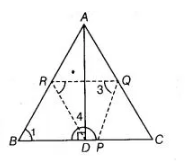# If P, Q and R are the mid-points of the sides,`
Question:

If $P, Q$ and $R$ are the mid-points of the sides, $B C, C A$ and $A B$ of a triangle and $A D$ is the perpendicular from $A$ on $B C$, then prove that $P, Q, R$ and $D$ are concyclic.

Solution:

Given in $\triangle A B C, P, Q$ and $R$ are the mid-points of the sides $B C, C A$ and $A B$ respectively Also, $A D \perp B C$. .

To prove $P, Q, R$ and $D$ are concyclic.

Construction Join $D R, R Q$ and $Q P$

Proof in right angled $\triangle A D P, R$ is the mid-point of $A B$.$\therefore \quad R B=R D$

$\Rightarrow$ $\angle 2=\angle 1$ ...(i)

[angles opposite to the equal sides are equal]

Since $B$ and $Q$ are the mid-points of $A B$ and $A C$, then

$R Q \| B C$ [by mid-point theorem]

or $R Q \| B P$

Since, $Q P \| R B$, then quadrilateral $B P Q R$ is a parallelogram.

$\Rightarrow$ $\angle 1=\angle 3$ ...(ii)

[opposite angles of parallelogram are equai]

From Eqs. (i) and (ii), $\angle 2=\angle 3$

But $\angle 2+\angle 4=180^{\circ}$ [linear pair axiom]

$\therefore$ $\angle 3+\angle 4=180^{\circ}$ $[\because \angle 2=\angle 3]$

Hence, quadrilateral $P Q R D$ is a cyclic quadrilateral. So, points $P, Q, R$ and $D$ are con-cyclic.

Hence proved.